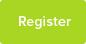# A Level Physics Exam Questions About Experiments

A summary of the key points to include when answering questions about experiments.

Date : 9/12/2017### Author Information

Subject : Physics

A Level Physics

Try to include most or all of the following points. This looks like a lot of work but most of these can be covered very briefly.

·       EQUIPMENT

o   Labelled diagram

o   List any equipment not shown in diagram

·       METHOD

o   What measurements do you need to take?

§  The actual physical measurements (i.e. diameter NOT cross-sectional area)

o   What equipment will you use to take each measurement?

§  Mention it, even if it’s something as obvious as using a ruler to measure length.

o   Range and values

§  E.g. “Load spring with 100g masses in 10 stages up to a maximum of 1kg.”

o   Repeats

§  Take 3 repeats and calculate mean if possible.

§  For small readings, measure in large multiples to reduce %-uncertainty (e.g. record time taken for 10 or 20 pendulum swings and then divide by 10 or 20 to calculate time period)

o   What calculations do you need to do?

§  (e.g. halve diameter to find radius then A =πr² for cross-sectional area)

·       RESULTS

o   This is nearly always a GRAPHICAL method, not just subbing one set of results into an equation.

o   What will you put on each axis?

§  Something you measured (e.g. current) or something you calculated from your measurements (e.g. resistance)

o   What do you need to read from the graph?

§  Intercept? (occasionally!)

§  Something else, like checking for a straight line through the origin (indicating proportionality)

o   Any further calculations?

§  E.g. Is your gradient the final “answer” or do you need to multiply/divide by a constant to get the value you’re looking for?

·       HEALTH & SAFETY

o   This doesn’t seem to be on the mark scheme so much these days but if there’s something obvious like wearing eye protection then mention it – can’t hurt!

This resource was uploaded by: Mark